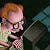# JavaScript Date Formatting Strings with D3

`date.ToString("MMM YYYY");`
• `%a` - abbreviated weekday name.
• `%A` - full weekday name.
• `%b` - abbreviated month name.
• `%B` - full month name.
• `%c` - date and time, as "%a %b %e %H:%M:%S %Y".
• `%d` - zero-padded day of the month as a decimal number [01,31].
• `%e` - space-padded day of the month as a decimal number [ 1,31]; equivalent to `%_d`.
• `%H` - hour (24-hour clock) as a decimal number [00,23].
• `%I` - hour (12-hour clock) as a decimal number [01,12].
• `%j` - day of the year as a decimal number [001,366].
• `%m` - month as a decimal number [01,12].
• `%M` - minute as a decimal number [00,59].
• `%L` - milliseconds as a decimal number [000, 999].
• `%p` - either AM or PM.
• `%S` - second as a decimal number [00,61].
• `%U` - week number of the year (Sunday as the first day of the week) as a decimal number [00,53].
• `%w` - weekday as a decimal number [0(Sunday),6].
• `%W` - week number of the year (Monday as the first day of the week) as a decimal number [00,53].
• `%x` - date, as "%m/%d/%Y".
• `%X` - time, as "%H:%M:%S".
• `%y` - year without century as a decimal number [00,99].
• `%Y` - year with century as a decimal number.
• `%Z` - time zone offset, such as "-0700".
• `%%` - a literal "%" character.

--

--## Steve Ellwood

Senior Integrations Officer at Doncaster Council Any views expressed are entirely my own.